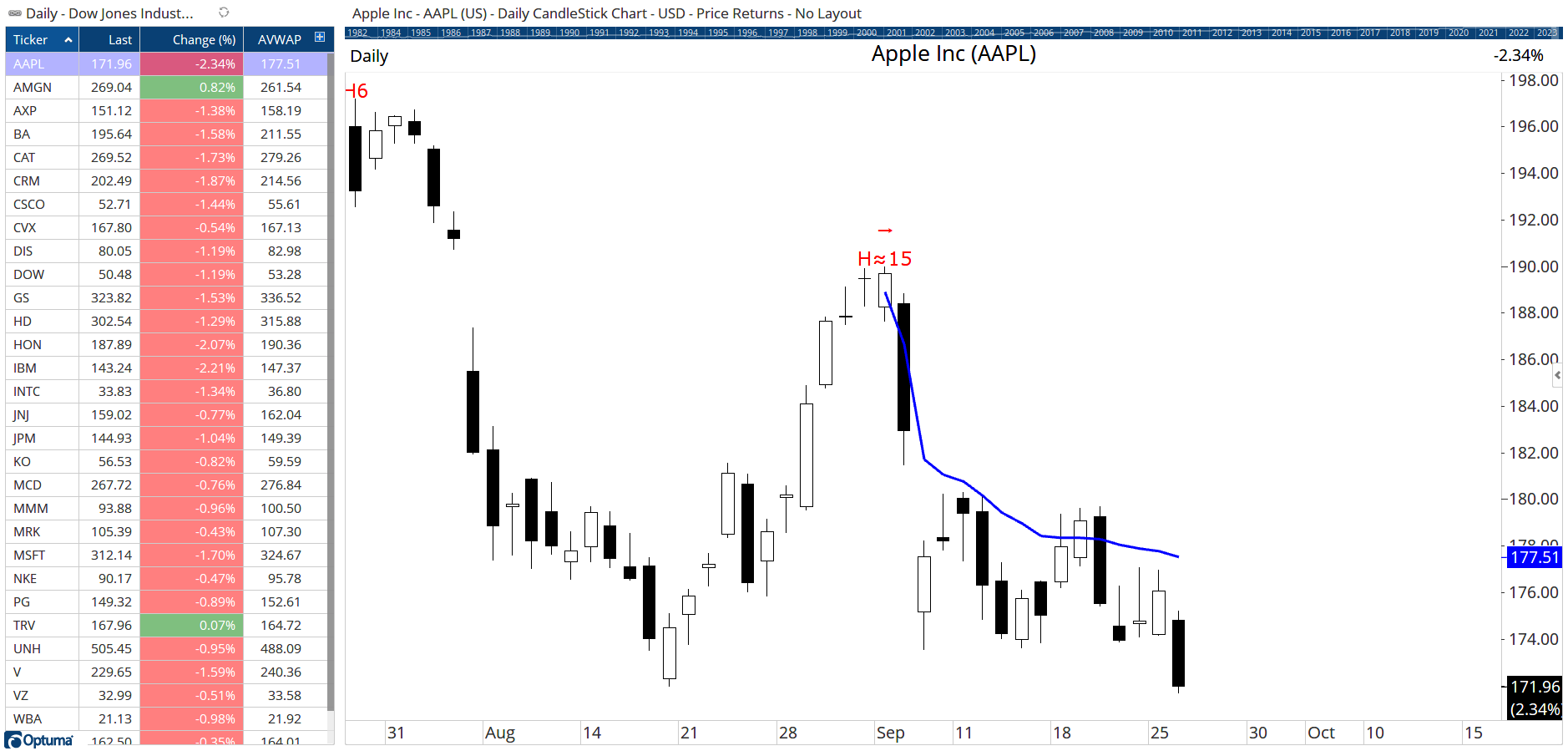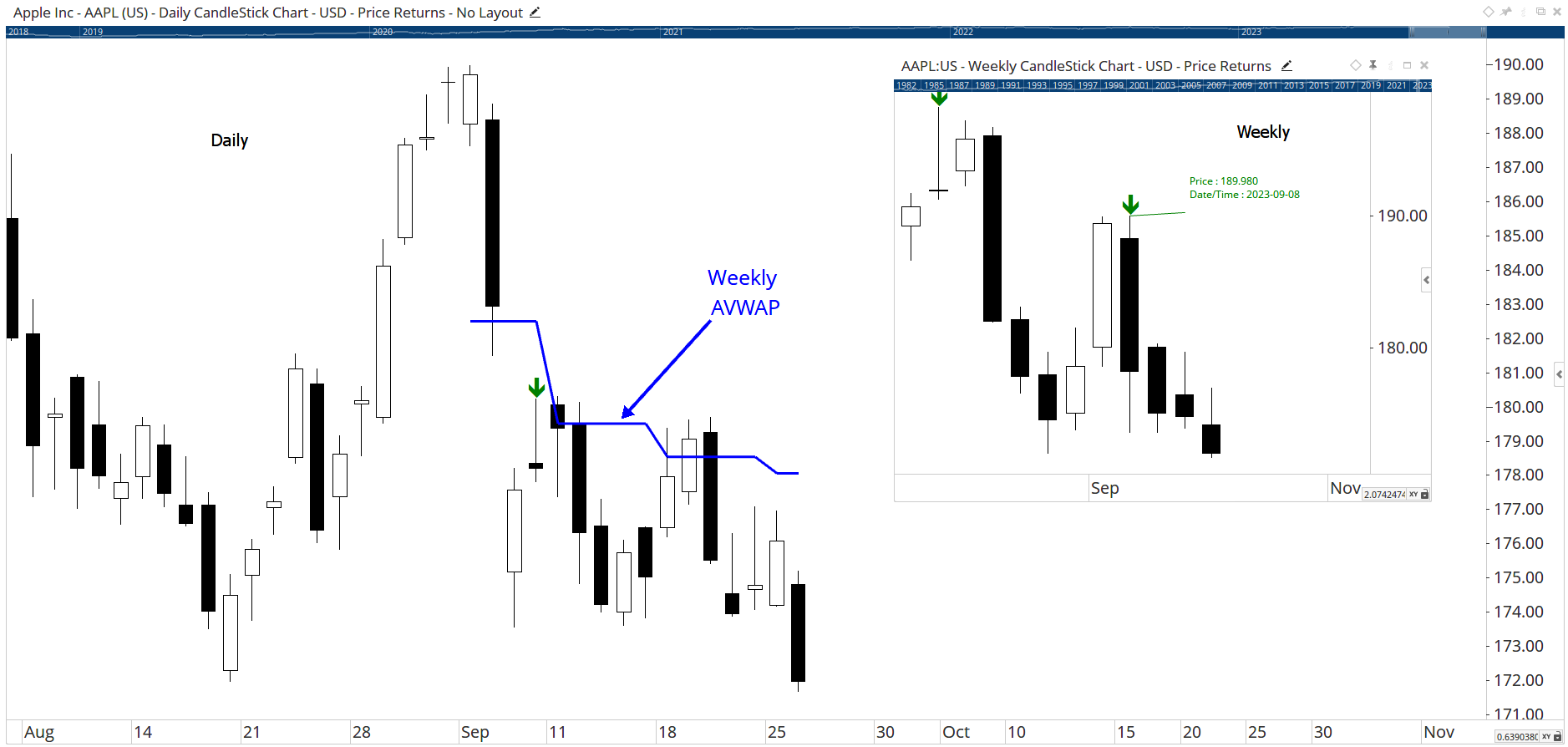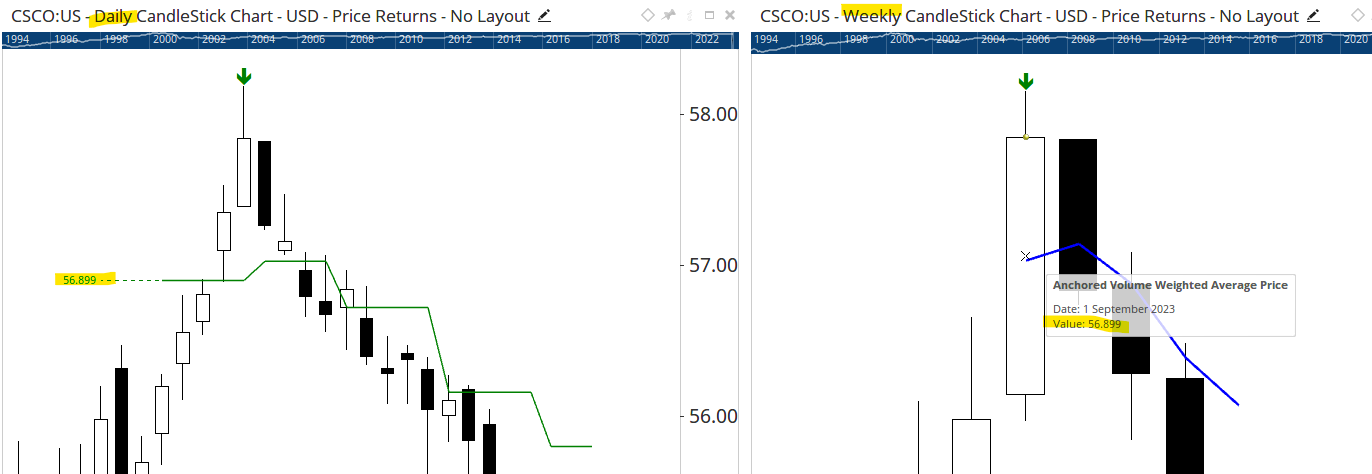Select Page

# Help with Weekly AVWAP on Daily Chart

Optuma Forums Optuma Scripting Help with Weekly AVWAP on Daily Chart

Viewing 6 posts - 1 through 6 (of 6 total)
• Author
Posts
• #71745

Hi. I was able to create AVWAP plot on Weekly Chart based off of a signal when Close crosses above a weekly High Pivot.
However, I have not been able to capture the same Weekly AVWAP on a Daily chart.
I suspect the issue is with using a Daily Date into AVWAP and not Weekly Date and maybe there is a way to convert the Weekly AVWAP line to a different
Anchored Plot that can be attached to a Daily Date on the Daily Chart.
Here is my script:

H = HIGH(Week(PERIODAMOUNT=1));
Prior_H = HIGH(Week(PERIODAMOUNT=1), OFFSET=1);
Next_H = HIGH(Week(PERIODAMOUNT=1), OFFSET=-1);
C = CLOSE(Week(PERIODAMOUNT=1));
cond1 = H > Prior_H;
cond2 = H > Next_H;
// Identify Weekly High Pivot based on cond1 and cond2
s1 = cond1 and cond2;
// Get Weekly High Price from High Pivot
v1 = VALUEWHEN(Week(PERIODAMOUNT=1), H, s1);
// Identify when Weekly Close Crosses Above Weekly High Pivot
s2 = C CrossesAbove v1;
// Get Weekly Bar Dates
v2 = BARDATE(Week(PERIODAMOUNT=1));
// Get Weekly Bar Dates for Weekly Close Crossing Above Weekly High Pivot
v3 = VALUEWHEN(Week(PERIODAMOUNT=1), v2, s2);
// Identify last occurrence of Weekly Close Crossing Above Weekly High Pivot
s3 = v2 == LAST(Week(PERIODAMOUNT=1), v3);
// Get Weekly Date of last occurrence of Weekly Close Crossing Above Weekly High Pivot
Weekly_Date = BarDate(Week(PERIODAMOUNT=1), NonZero(s3));
// Get Daily Bar Dates
v4=BARDATE(Day(PERIODAMOUNT=1));
// Identify Daily Bar Date that has the same Weekly Date
s4 = v4==Weekly_Date;
// Get Daily Date of Daily Bar that has the same Weekly Date
\$Daily_Date = BarDate(Day(PERIODAMOUNT=1), NonZero(s4));

Plot1 = AVWAP(Week(PERIODAMOUNT=1), BACKTYPE=Fixed, DATE=\$Daily_Date, CALC=HLC);

#71747

Hi Dan,

Yes the daily bardate would not match the weekly bardate (unless it occurred on a Friday).

Did you see this AVWAP pivot script?

https://forum.optuma.com/topic/anchored-vwap-scripts/#post-70977

It’s not based on weekly data but if the Pivot Label value (\$PL in the formula) is changed to 5 then it will require at least 5 days either side of the high to confirm the high, and the Show Plot will automatically draw the AVWAP from there. Here’s an example of AAPL from the recent pivot high (which occurred 15 days ago):#71749

However, the question is, can AVWAP with weekly timeframe be linked to a specific Date on a Daily Chart?
For example, if I get the Weekly AVWAP from past week’s Date: 2023-09-22, can I then somehow link it to the Daily Bar that has the same Date:  2023-09-22?

v1 = AVWAP(Week(PERIODAMOUNT=1), BACKTYPE=Fixed, DATE=2023-09-22, CALC=HLC)

The goal is to try to use AVWAP as actual past Week Support/Resistance on a Daily Chart.

#71751

OK thanks. If I’m understanding correctly the weekly pivot is when the highs either side of a weekly bar are lower. We can script this with TurnsDown as follows, and then plot the weekly AVWAP from that week:

So in the example of AAPL, the last weekly high pivot was on Sept 8th, with the weekly AVWAP plotted on the daily chart in blue from that week:#71753

Thanks. I am with you so far and was able to accomplish the same thing on a Weekly Chart.
Now, how can I can I further script it so that the same AVWAP Weekly calculation (AVWAP(Week(PERIODAMOUNT=1), BACKTYPE=Fixed, DATE=\$DATE) you are using can be now used in a Daily Chart with the same Daily Date as the Weekly Date?
I am hoping to using AVWAP to measure Daily support/resistance of off a higher timeframe which is Weekly in this case.
So I am hoping I can run the script in a Daily Chart.
If you understand my goal, can this be achieved somehow?

#71755

On a daily chart it will be drawn on the same week as the weekly signal, but it will be a horizontal line for that week beginning on the Monday, which is why it appears stepped.

In the example of CSCO below, the weekly signal was Sept 1st (all weekly data is dated as of the Friday). If you put the AVWAP tool on the weekly chart it will have a value of 56.899 for that week, which matches the Show Plot tool on the daily chart – it just begins on the Monday of that week even though the final value of that week would not have been known until the Friday (there’s no way around that when using weekly data).

Hope that makes sense, but it’s one of the pitfalls of mixing timeframe signals.Viewing 6 posts - 1 through 6 (of 6 total)
• You must be logged in to reply to this topic.